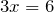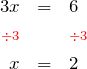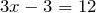# Category: Algebra

In an earlier post, we looked at expanding binomial products. Remember how the result of the expansion is a mixture of the inputs — the numbers in the input do not appear in the result, but the numbers that …

## Solving Equations

A typical maths textbook presents equation solving in a very ordered, structured way. Starting with one-step equations such as, they introduce the single step solution processbefore moving onto two-step equations such as. For these equations …

## Expanding Binomial Products

Welcome to the first mathematical post in The Mathenæum Blog. In this post, I have chosen to discuss expanding binomial products, because in many ways binomial products act as a bridge between elementary algebraic work, and more advanced applications …